## FSA, Week5: Fast Modular Arithmetic and Public Key Cryptography

Jan van Eijck

October 4, 2016

> module FSA5 where
>
> import Data.List
> import System.Random

Abstract

Fast modular arithmetic allows us to generate and recognize large primes efficiently, but we need the concept of a probabilistic algorithm.

Given two large primes $$p$$ and $$q$$, computing their product $$pq$$ is easy, but finding the two factors from the semi-prime $$pq$$ is (believed to be) hard. No fast algorithm for this is known.

These facts have important consequences for cryptography. The lecture gives an introduction to fast modular arithmetic, to probabilistic algorithms for primality testing, and to their application in public key cryptography.

Background

• Chapter 33 of (Cormen, Leiserson, and Rivest 1997), on number-theoretic algorithms.

• Fast primality testing (Miller 1976),(Rabin 1980).

• Cryptographic key exchange (Diffie and Hellman 1976).

• RSA public key cryptography (R. Rivest, Shamir, and Adleman 1978).

Factorization

Here is a naive factorization algorithm:

> factorsNaive :: Integer -> [Integer]
> factorsNaive n0 = factors' n0 2 where
>   factors' 1 _ = []
>   factors' n m
>     | n mod m == 0 = m : factors' (n div m) m
>     | otherwise      =     factors' n (m+1)

This gives:

 *FSA5> factorsNaive 362880
[2,2,2,2,2,2,2,3,3,3,3,5,7]
*FSA5> factorsNaive 524287


Improvement

This can be improved slightly by only trying candidate factors that are primes:

> factors :: Integer -> [Integer]
> factors n0 = let
>    ps = takeWhile (\m -> m^2 <= n0) primes
>  in factors' n0 ps where
>    factors' 1 _  = []
>    factors' n [] = [n]
>    factors' n (p:ps)
>     | n mod p == 0 = p: factors' (n div p) (p:ps)
>     | otherwise      =    factors' n ps

To implement primes we can use factors itself:

> prime :: Integer -> Bool
> prime n = factors n == [n]
> primes :: [Integer]
> primes = 2 : filter prime [3..]

Comparison

To compare the two versions, try this out with:

 *FSA5> map factors [m8..]

*FSA5> map factorsNaive [m8..]

Here m8 is the eighth Mersenne prime (see below).

Despite this improvement, it is generally believed that factorization of numbers with large factors is hard.

Unsolved problem in computer science

Can integer factorization be done in polynomial time?

No efficient algorithm for factorization is known. All existing methods use trial division with large numbers of candidates.

Hardest instances: semiprimes (products of two prime numbers).

Look up Integer Factorization

Primality Testing Using Factorization

The test

  prime n = factors n == [n]

is 100 percent reliable but inefficient, because it is essentially based on trial and error.

The question is: can we do better?

It turns out that for primality testing we can, but it seems that for factorization we cannot.

These facts have important implications for cryptography, as we will explain in today's lecture.

Mersenne Primes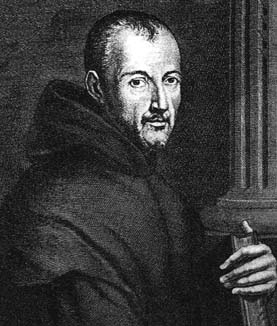Marin Mersenne (1588--1647)

Father Marin Mersenne was a French priest and amateur mathematician. He discovered some large primes by studying numbers of the form $$2^p - 1$$. Such primes are called Mersenne primes.

The first 25 Mersenne primes

> mers :: Integer -> Integer
> mers 1  = 2^2-1;    mers 2  = 2^3-1;     mers 3  = 2^5-1
> mers 4  = 2^7-1;    mers 5  = 2^13-1;    mers 6  = 2^17-1
> mers 7  = 2^19-1;   mers 8  = 2^31-1;    mers 9  = 2^61-1
> mers 10 = 2^89-1;   mers 11 = 2^107-1;   mers 12 = 2^127-1
> mers 13 = 2^521-1;  mers 14 = 2^607-1;   mers 15 = 2^1279-1
> mers 16 = 2^2203-1; mers 17 = 2^2281-1;  mers 18 = 2^3217-1
> mers 19 = 2^4253-1; mers 20 = 2^4423-1;  mers 21 = 2^9689-1
> mers 22 = 2^9941-1; mers 23 = 2^11213-1; mers 24 = 2^19937-1
> mers 25 = 2^21701-1;
> mers _  = undefined

Print out mers 25 to see that it is a huge beast.

$$2^n-1$$ can only be prime if $$n$$ is also prime (Exercise 3.36 in (Doets and Eijck 2012)).

Suppose $$n$$ composite, i.e., let $$n = ab$$.

Let $$x = 2^b -1$$ and let $$y = 1 + 2^b + 2^{2b} + \cdots + 2^{(a-1)b}$$.

Then $$2^b \cdot y = 2^b + 2^{2b} + \cdots + 2^{ab}$$.

Therefore $$xy = (2^b - 1)y = 2^b \cdot y - y = 2^{ab} - 1$$.

This shows that $$2^n - 1$$ is composite.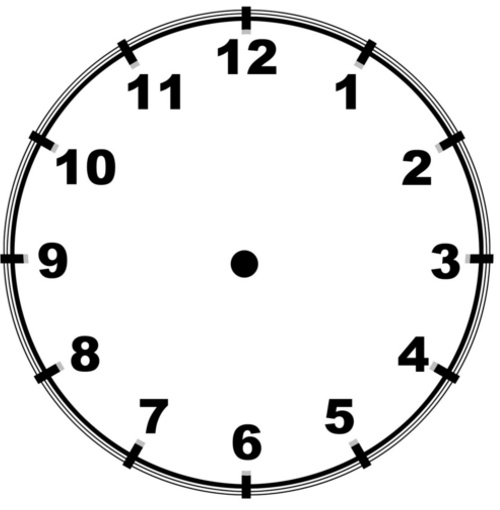Modular Arithmetic

$11 + 4 = 15 \equiv 3 \pmod {12}.$

Operations for modular addition, multiplication

Modular addition:

> addM :: Integer -> Integer -> Integer -> Integer
> addM x y = rem (x+y)

Modular multiplication:

> multM :: Integer -> Integer -> Integer -> Integer
> multM x y = rem (x*y) 

Modular Inverses: Example $${\mathbb Z}/5$$.

• Equivalence classes are $$, , , , $$.

• Multiplication satisfies $$[a] \cdot [ b ] = [a \cdot b]$$.

• $$ \cdot  = $$.

• $$ \cdot  =  = $$.

• $$ \cdot  =  = $$.

• $$ \cdot  =  = $$.

• So every class except $$$$ has a multiplicative inverse.

• Compare $${\mathbb Z}$$: only $$1$$ and $$-1$$ have multiplicative inverses.

In arithmetic mod $$n$$, $$[a]$$ has a multiplicative inverse $$[b]$$ if $$[a] \cdot [b] = $$.

This means that $$[a \cdot b] = $$ where

$$ = \{ \ldots, 1 - 2n, 1 - n , 1, 1 + n , 1 + 2n, \ldots \}$$.

This means in turn that $$ab = 1 + kn$$ for some integer $$k$$.

This is the case iff $$ab - kn = 1$$, and this is only possible if $$n$$ and $$a$$ do not have a common divisor.

Modular division, modular inverse

Modular division is the same as multiplication by modular inverse. The modular inverse of $$x$$ exists if and only if $$x$$ is co-prime with its modulus.

Indeed, $$\gcd (x, n) = 1$$ iff (extended Euclidean algorithm) there are $$u,v$$ with $$ux + vn = 1$$ iff there is $$u$$ with $$ux \equiv 1 \bmod n$$.

Thus, the following function gives us modular inverses:

> invM :: Integer -> Integer -> Integer
> invM x n = let
>    (u,v) = fctGcd x n
>    copr  = x*u + v*n == 1
>    i     = if signum u == 1 then u else u + n
>  in
>    if copr then i else error "no inverse"

This uses the extended Euclidean algorithm for GCD.

The Extended Euclidean Algorithm

> fctGcd :: Integer -> Integer -> (Integer,Integer)
> fctGcd a b =
>   if b == 0
>   then (1,0)
>   else
>      let
>        (q,r) = quotRem a b
>        (s,t) = fctGcd b r
>      in (t, s - q*t)

Extended Euclid: Algebraic Version

You can think of the extended Euclidean Algorithm as an algebraic version of the Euclidean Algorithm. Instead of computing the result of (say) subtracting $$b$$ from $$a$$ some number of times (say $$y$$ times), we now compute $$\gcd (a,b) = \gcd (a -yb, b)$$, and so on.

Correctness of the extended Euclidean algorithm

1. Suppose $$b = 0$$. Then the gcd of $$a$$ and $$b$$ is $$a$$, and indeed $$a = 1a + 0b$$.

2. Suppose $$b > 0$$. Assume that for $$0 \leq m < n \leq b$$ it holds that $$\text{fctGcd} \ n \ m$$ yields $$(x,y)$$ with $$\gcd(n,m) = xn + ym$$. Now let $$q,r$$ be such that $$a = qb + r$$. We may suppose, by the induction hypothesis, that $$\text{fctGcd} \ b \ r$$ yields $$(s,t)$$ with $$\gcd (b, r) = sb + tr$$. From $$a = qb + r$$ we get that $$r = a - qb$$. Since $$\gcd ( a , b) = \gcd (b, r)$$ we have that $$\gcd ( a , b) = \gcd (b , r) = sb + t(a - qb) = ta + (s - qt)b$$. So $$t$$ and $$(s-qt)$$ are the required integers. QED

Coprimes

> coprime :: Integer -> Integer -> Bool
> coprime n m = gcd n m == 1

Another version:

> coprime' :: Integer -> Integer -> Bool
> coprime' n m = let (x,y) = fctGcd n m
>                in x*n + y*m == 1

Aside: Running the Euclidean Algorithm Backwards

Define trees, as follows:

> data Tree a = T a [Tree a] deriving (Eq,Ord,Show)

Growing a tree:

> grow :: (node -> [node]) -> node -> Tree node
> grow step seed = T seed (map (grow step) (step seed))

Taking a finite part of a (possibly infinite) tree:

> takeT :: Int -> Tree a -> Tree a
> takeT 0 (T x _) = T x []
> takeT n (T x ts) = T x (map (takeT (n-1)) ts)

Example:

 *FSA5> takeT 2 $grow (\ x -> [x+1,x+1]) 0 T 0 [T 1 [T 2 [],T 2 []],T 1 [T 2 [],T 2 []]] Running Euclid Backwards > coprimeT :: Tree (Integer,Integer) > coprimeT = grow f (1,1)  > f :: (Integer,Integer) -> [(Integer,Integer)] > f (n,m) = [(n+m,m),(n,n+m)]  *FSA5> takeT 2 coprimeT T (1,1) [T (2,1) [T (3,1) [],T (2,3) []],T (1,2) [T (3,2) [],T (1,3) []]] Collecting the coprimes > pairs :: [(Integer,Integer)] > pairs = concatMap (\ n -> zip [1..n] (repeat n)) [1..] > coprimes :: [(Integer,Integer)] > coprimes = filter (uncurry coprime) pairs Modular Exponentiation > expM :: Integer -> Integer -> Integer -> Integer > expM x y = rem (x^y) This is not efficient, for we first compute $$x^y$$, and then reduce the result modulo $$N$$. Instead, we should have performed the intermediate computation steps for $$x^y$$ modulo $$N$$. Modular Exponentiation, Fast Version One of your exercises for this week's lab session is to implement a function that does modular exponentiation of $$x^y$$ in polynomial time, by repeatedly squaring modulo $$N$$. E.g., $$x^{33} \bmod 5$$ can be computed by means of $x^{33} \bmod 5 = x^{32} \bmod 5 \times x \bmod 5.$ $$x^{32} \pmod N$$ is computed in five steps by means of repeatedly squaring modulo $$N$$: $[x] \rightarrow [x^2] \rightarrow [x^4] \rightarrow [x^8] \rightarrow [x^{16}] \rightarrow [x^{32}].$ Task for you > exM :: Integer -> Integer -> Integer -> Integer > exM = expM -- to be replaced by a fast version Fermat's Test for PrimalityPrimality testing using a probabilistic algorithm is based on efficient exponentiation modulo. This uses a theorem by the famous French mathematician Pierre de Fermat (1601--1665). Fermat's Little Theorem The following is called the Little Theorem of Fermat, to distinguish it from Fermat's famous Last Theorem. (Fermat's Last Theorem says that $$x^n + y^n = z^n$$ has no non-zero integer solutions for natural numbers $$n> 2$$. Fermat had stated this without proof. A proof was found by Andrew Wiles in 1995.) Fermat: If $$p$$ is prime, then for every integer $$a$$ with $$1 \leq a < p$$: $$a^{p-1} \equiv 1 \pmod p$$. Some Examples To see what Fermat's Little Theorem says, look at some examples: Assume $$p = 5$$. Let us check $$2^4$$, $$3^4$$ and $$4^4$$. $[2^4] =  = , [3^4] =  = , [4^4] =  = .$ Next, use Haskell to check for a larger prime:  *FSA5> [ a^28 mod 29 | a <- [1..28] ] [1,1,1,1,1,1,1,1,1,1,1,1,1,1,1,1,1,1,1,1,1,1,1,1,1,1,1,1] Consider the list $$[ 1, \ldots, p-1 ]$$. These are the remainders modulo $$p$$ of all $$a$$ with $$1 \leq a < p$$. For any $$a$$ with $$1 \leq a < p$$, multiplying the elements of the list $$[ 1, \ldots, p-1 ]$$ with $$a$$ modulo $$p$$ is simply to permute the list. Let's try this out with Haskell:  Prelude FSA5> [n mod 13 | n <- [1..12] ] [1,2,3,4,5,6,7,8,9,10,11,12] Prelude FSA5> [2*n mod 13 | n <- [1..12] ] [2,4,6,8,10,12,1,3,5,7,9,11] Prelude FSA5> [3*n mod 13 | n <- [1..12] ] [3,6,9,12,2,5,8,11,1,4,7,10] Prelude FSA5> [4*n mod 13 | n <- [1..12] ] [4,8,12,3,7,11,2,6,10,1,5,9] Prelude FSA5> [5*n mod 13 | n <- [1..12] ] [5,10,2,7,12,4,9,1,6,11,3,8] Prelude FSA5> [6*n mod 13 | n <- [1..12] ] [6,12,5,11,4,10,3,9,2,8,1,7] Prelude FSA5> [7*n mod 13 | n <- [1..12] ] [7,1,8,2,9,3,10,4,11,5,12,6] Prelude FSA5> [8*n mod 13 | n <- [1..12] ] [8,3,11,6,1,9,4,12,7,2,10,5] Prelude FSA5> [9*n mod 13 | n <- [1..12] ] [9,5,1,10,6,2,11,7,3,12,8,4] Take the case with $$p = 13$$. Multiplying the numbers in the list $$[ 1, \ldots, 12 ]$$ and the numbers in the list $$[ 5n \bmod 13 \mid n \in \{ 1, \ldots, 12 \} ]$$ we get the same outcome:  *FSA5> product [1..12] 479001600 *FSA5> product (map (\ n -> 5*n mod 13) [1..12]) 479001600 Dividing both sides by $$12!$$, this gives $$5^{12} \equiv 1 \bmod 13$$. Compare the lists $$[, \ldots, [p -1]]$$ and $$[[a], [2a], \ldots, [(p-1)a]]$$. Suppose $$[[a], [2a], \ldots, [(p-1)a]]$$ is a permutation of $$[, \ldots, [p -1]]$$ Then we can take the product on both sides: $$(p -1)! = a^{p-1} (p -1)! \mod p$$. Divide on both sides by $$(p-1)!$$: $$1 = a^{p-1} \mod p$$. So if we can prove that $$[[a], [2a], \ldots, [(p-1)a]]$$ is a permutation of $$[, \ldots, [p -1]]$$ we are done and we have proved Fermat's Little Theorem. Fermat's Little Theorem: Proof Now for the general case. We have to show that if the numbers in the set $S = \{ 1, \ldots, p-1 \}$ get multiplied by $$a$$ modulo $$p$$, the resulting numbers are distinct and $$\neq 0$$. So let $$i \neq j \in S$$, and consider $$ai$$ and $$aj$$. Suppose $$ai \equiv aj \pmod p$$. Then $$i \equiv j \pmod p$$ and contradiction with $$i \neq j$$. So $$ai$$ and $$aj$$ are distinct modulo $$p$$. If $$ai \equiv 0$$ then, since $$a$$ and $$p$$ are relatively prime, we can divide by $$a$$ and get $$i \equiv 0$$, and contradiction. So the resulting numbers are $$\neq 0$$. This means the result of multiplying the numbers in $$S$$ with $$a$$ modulo $$p$$ is a permutation of $$S$$. This gives $S = \{ 1, \ldots, p-1 \}$ $= \{ a \times 1 \bmod{p}, \ldots, a \times p-1 \bmod{p} \}.$ Multiplying the numbers left and right gives: $(p-1)! = a^{p-1} \times (p-1)! \pmod{p}.$ We can divide both sides by $$(p-1)!$$ because $$(p-1)!$$ and $$p$$ are relatively prime. This gives $$a^{p-1} \equiv 1 \pmod{p}$$. QED Finite Fields or Galois Fields A field is a set that is closed under multiplication, division, addition and subtraction. Examples are the rationals and the reals, but there are also finite examples. The most well known example is the field of integers modulo a prime number $$p$$, $$GF(p)$$. Note that for every $$x,y$$ in $$GF(p)$$ it holds that $$(x + y)^p = x^p + y^p$$. This is because, by Newton's binomium: $(x + y)^p = \sum_{k = 0}^{p} \binom{p}{k} x^k y^{p-k} \equiv x^p + y^p \pmod{p}$ since $$\binom{p}{k}$$ has a factor $$p$$ for all $$k$$ with $$0 < k < p$$. Also, it follows from Fermat's little theorem that $$x^p \equiv x \pmod{p}$$ for all $$x$$. Fermat's little theorem can be restated as: every nonzero element $$x$$ of $$GF(p)$$ is a root of unity, i.e., $$x^{p-1} = 1$$. Fermat's Algorithm for Primality Testing • Pick $$a$$ with $$1 < a < N$$ at random. • Compute $$a^{N-1} \pmod N$$ using fast exponentiation. • If the outcome is $$1$$, • output "Probably Prime", • otherwise output "Composite". If $$N$$ is indeed prime then $$a^{N-1} \equiv 1 \pmod N$$, and the test works fine. But if $$N$$ is composite, it may still happen that $$a^{N-1} \equiv 1 \pmod N$$, for Fermat's Little Theorem does not specify what happens for composite numbers. Implementation Fermat's algorithm yields the following primality test: > primeTestF :: Integer -> IO Bool > primeTestF n = do > a <- randomRIO (2, n-1) :: IO Integer > return (exM a (n-1) n == 1) Improving the Fermat Algorithm A better prime test results if we try out more candidates for $$a$$: > primeTestsF :: Int -> Integer -> IO Bool > primeTestsF k n = do > as <- sequence$ fmap (\_-> randomRIO (2,n-1)) [1..k]
>  return (all (\ a -> exM a (n-1) n == 1) as)

There remains a possibility of getting false positives

One of your tasks for this week will be to test this function.

An Addition to the Fermat Test: Miller and Rabin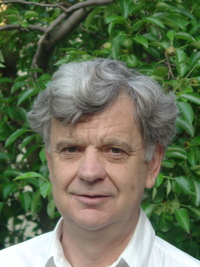Gary Miller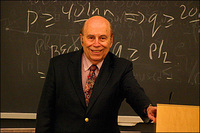Michael Rabin

Unfortunately, there are numbers that can fool the Fermat test, so called Carmichael numbers. You will encounter Carmichael numbers in the lab exercises.

Miller and Rabin (Miller 1976), (Rabin 1980) propose to add a further test, called the Miller-Rabin primality test.

Lemma: If $$n$$ is a prime, then any number $$x$$ with the property that $$x^2 = 1 \bmod n$$ has to satisfy $$x = 1 \bmod n$$ or $$x = -1 \bmod n$$.

Proof: From $$x^2 = 1 \bmod n$$ it follows that $$x^2 - 1 = (x-1)(x+1) = 0 \bmod n$$. This means that $$n$$ divides $$(x-1)(x+1)$$. Since $$n$$ is prime, it follows from this (by a lemma called Euclid's Lemma) that $$n$$ has to divide either $$x-1$$ or $$x+1$$. In the former case we have $$x-1 = 0 \bmod n$$, i.e., $$x = 1 \bmod n$$. Int the latter case we have $$x = -1 \bmod n$$. QED

Note that $$-1 = n-1 \bmod n$$.

Euclid's Lemma: If $$p$$ is prime and $$p \mid ab$$ then either $$p \mid a$$ or $$p \mid b$$.

Proof of Euclid's Lemma:

Observe that $$p \not\,\mid a$$ implies that $$p$$ and $$a$$ are co-prime.

So suppose $$p \mid ab$$ and $$p \not\,\mid a$$. Then, by Euclid's extended GCD algorithm, there are $$x,y \in {\mathbb Z}$$ with $$xa + yp = \gcd(a,p) = 1$$. Multiplying both sides by $$b$$ gives $$xab + ypb = b$$. Since it is given that $$p \mid ab$$ we get that $$p \mid b$$. QED

• Let's entertain the hypothesis that $$n$$ is prime.

• Then by Fermat's Little Theorem, for any $$a$$ with $$1 \leq a < n$$: $$a^{n-1} = 1 \mod n$$.

• We may assume $$n-1$$ is even, so $$n-1 = 2s$$, and $$a^{2s} = 1 \mod n$$.

• By the theorem above, $$a^s = 1 \mod n$$ or $$a^s = -1 \mod n$$.

• In the former case, suppose $$s = 2t$$. Then $$a^{2t} = 1 \mod n$$.

• By the theorem above, $$a^t = 1 \mod n$$ or $$a^t = -1 \mod n$$.

• And so on

Factoring out powers of 2

The following function factors out powers of 2:

> decomp :: Integer -> (Integer,Integer)
> decomp n = decomp' (0,n) where
>   decomp' = until (odd.snd) (\ (m,n) -> (m+1,div n 2))

This gives:

*FSA5> decomp 53248
(12,13)
*FSA5> 2^12 * 13
53248

The Miller-Rabin Primality Test

The first argument gives the number of trials.

> primeMR :: Int -> Integer -> IO Bool
> primeMR _ 2 = return True
> primeMR 0 _ = return True
> primeMR k n = error "not yet implemented"

Testing the Primality Tests

For testing our primality test algorithms the list of prime numbers generated by Eratosthenes' sieve is useless, for the algorithms all correctly classify the primes as primes. Where they can go wrong is on classifying composite numbers; these can slip through the Fermat test, and also through the Rabin/Miller test, although the probability of this can be made arbitrarily small.

The composite numbers are given by:

> composites :: [Integer]
> composites = error "not yet implemented"

Application to Cryptography

The idea to base public key cryptography on the fact that finding large primes, multiplying them, and exponentiation modulo a prime are easy while factoring numbers that are multiples of large primes, and finding $$x$$ from $$x^a \bmod p$$ (taking the discrete logarithm) are hard was put forward in (Diffie and Hellman 1976).Whitfield Diffie and Martin Hellman

Diffie-Hellman Key Exchange Protocol

• Alice and Bob agree on a large prime $$p$$ and a base $$g < p$$ such that $$g$$ and $$p-1$$ are co-prime.

• Alice picks secret $$a$$, sends $$g^a \bmod p= A$$ to Bob.

• Bob picks secret $$b$$, sends $$g^b \bmod p = B$$ to Alice.

• Alice calculates $$k = B^a \bmod p$$.

• Bob calculates $$k = A^b \bmod p$$.

• They now share key $$k$$, for $$k = (g^a)^b = (g^b)^a \bmod p$$.

Use of a Shared Secret Key for Secure Communication

Let $$p$$ be the prime that Alice and Bob have agreed on, and let $$k$$ be their shared key. Then message $$m$$ is encoded as

$m \times k \bmod p.$

> encodeDH :: Integer -> Integer -> Integer -> Integer
> encodeDH p k m = error "not yet implemented" 

Such messages can be decoded by both Alice and Bob.

Alice knows $$p$$, $$k$$, $$g^b$$ and $$a$$. She decodes cipher $$c$$ with

$c \times (g^b)^{(p-1)-a} \bmod p.$

Bob knows $$p$$, $$k$$, $$g^a$$ and $$b$$. He decodes cipher $$c$$ with

$c \times (g^a)^{(p-1)-b} \bmod p.$

What is behind this is again Fermat's Little Theorem

We have:

$(g^a)^{(p-1)-b} = g^{a((p-1)-b)} = g^{a(p-1)} \cdot g^{-ab} = (g^{p-1})^a \cdot g^{-ab}$

$\stackrel{Fermat}{=} 1^a \cdot g^{-ab} = g^{-ab} \bmod p,$

and therefore:

$c \cdot (g^a)^{(p-1)-b} = (m \cdot g^{ab}) \cdot g^{-ab} =$

$= m \cdot (g^{ab} \cdot g^{-ab}) = m \bmod p.$

This gives:

First argument is the prime, second argument the key, third argument message A, fourth argument the secret of b and final argument the code.

> decodeDH :: Integer -> Integer -> Integer
>          -> Integer -> Integer -> Integer
> decodeDH p k ga b c = error "not yet implemented"

Symmetric Key Cyphers

This is called a symmetric key cypher.

Alice and Bob use the same key to encrypt, and a small variation on this key, together with the private information they have, to decrypt.

Symmetric Key Cyphers Using Fast Exponentiation Modulo

> encode :: Integer -> Integer -> Integer -> Integer
> encode p k m = let
>    p' = p-1
>    e  = head [ x | x <- [k..], gcd x p' == 1 ]
>  in
>    exM m e p
>
> decode :: Integer -> Integer -> Integer -> Integer
> decode p k m = let
>    p' = p-1
>    e  = head [ x | x <- [k..], gcd x p' == 1 ]
>    d  = invM e p'
>  in
>    exM m d p

Efficient With Key, Hard Without

Finding appropriate $$e$$ and $$d$$ for coding and decoding when $$p$$ and $$k$$ are known is fast.

Suppose one were allowed to keep the modulus $$p$$ and the lower bound for the exponent $$k$$ hidden in the above coding function. Then $$p,k$$ is the key to both coding and decoding.

If $$p,k$$ are both known, coding and decoding are both easy (see above). If $$p,k$$ are unknown, both coding and decoding are hard.

> cipher :: Integer -> Integer
> cipher = encode secret bound
> decipher :: Integer -> Integer
> decipher = decode secret bound

Look at this

 *FSA5> cipher 123
59919695618995421503916756397503286001898487947920162937
23993242822009438133642886682530069654361563275429068325
99325957585106515246102899061628169372657254294820399394
78144012585334805003984324282240871591536702957793570769
96956357790457581539952685957650593709298723348227

*FSA5> decipher 599196956189954215039167563975032860018
98487947920162937239932428220094381336428866825300696543
61563275429068325993259575851065152461028990616281693726
57254294820399394781440125853348050039843242822408715915
36702957793570769969563577904575815399526859576505937092
98723348227
123

A Problem

There is a problem, however. In order to construct the function cipher, the secret prime and the bound for the exponent have to be available, so we cannot make the full recipe for constructing the encoding function publicly available.

This can be solved by a variation on the Diffie-Hellman key exchange protocol.

Aside: Euler's totient function

Euler's totient function counts the positive integers $$k$$ up to a given integer $$n$$ that are coprime with $$n$$.

Implementation:

> totient :: Integer -> Integer
> totient n = toInteger \$ length [ k | k <- [1..n], gcd k n == 1 ]

If we know that the input integer is a semiprime (product of primes $$p$$ and $$q$$) then we have for the totient function:

> phi :: Integer -> Integer -> Integer
> phi p q = (p - 1) * (q - 1)

Asymmetric Public Key Cryptography

In asymmetric public key cryptography, it turns out we can do much better than this.

We can generate a key and make it publicly available, and from that key anyone can construct an encoding function.

Decoding, given only this public key information, is generally thought to be hard.

RSA = Rivest, Shamir, AdlemanLen Adleman -- Adi Shamir -- Ron Rivest

See (R. Rivest, Shamir, and Adleman 1978).

RSA public key cryptography; generation of a public key

If we have two large primes $$p,q$$ we select an integer that is coprime with the totient for encoding.

> select :: Integer -> Integer -> Integer
> select p q = let
>    t = phi p q
>  in
>    head [ x | x <- [3..], gcd x t == 1 ]
> rsaPublic :: Integer -> Integer -> (Integer,Integer)
> rsaPublic p q = error "not yet implemented" 

Here $$p$$ and $$q$$ are supposed to be large primes with the same bitlength.

RSA public key cryptography; generation of a private key

Let $$p,q$$ be large primes. Let $$n = pq$$.

If $$(e,n)$$ is the public key pair, where $$n$$ is the semiprime given by $$n = pq$$, and $$d$$ is the inverse of $$e$$ modulo $$(p-1)(q-1)$$, then $$(d,n)$$ is the private key.

It is believed that the private key is hard to compute from the public key (in the sense that nobody knows how to do this, and it is suspected that it is impossible to find an efficient algorithm for this).

> rsaPrivate ::  Integer -> Integer -> (Integer,Integer)
> rsaPrivate p q = error "not yet implemented"

RSA encoding using a generated key pair

rsaEncode should use a public key pair to encode a message (represented by an integer).

> rsaEncode :: (Integer,Integer) -> Integer -> Integer
> rsaEncode (e,n) m =  error "not yet implemented" 

RSA decoding using a private key

RSA decoding using a private key is just a matter of using the private key to encode again, so we have:

> rsaDecode = rsaEncode                              

Why does this work?

$(m^e)^{e^{-1}} \bmod n = m.$

A genuine trapdoor function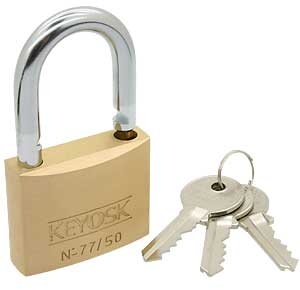padlock

> trapdoor :: (Integer,Integer) -> Integer -> Integer
> trapdoor = rsaEncode 

Toy example

  *FSA5> rsaPublic 13 17
(5,221)
*FSA5> rsaPrivate 13 17
(77,221)
*FSA5> rsaEncode (5,221) 20
141
*FSA5> rsaDecode (77,221) 141
20

Links

Lab work week 5

Back to main course page

> secret, bound :: Integer
> secret = mers 18
> bound  = 131

Cormen, Thomas H., Charles E. Leiserson, and Ronald L. Rivest. 1997. Introduction to Algorithms. MIT Press.

Diffie, W., and M. Hellman. 1976. “New Directions in Cryptography.” IEEE Transactions on Information Theory 22 (6): 644–54.

Doets, K., and J. van Eijck. 2012. The Haskell Road to Logic, Maths and Programming, Second Edition. Vol. 4. Texts in Computing. London: College Publications.

Miller, Gary L. 1976. “Riemann’s Hypothesis and Tests for Primality.” Journal of Computer and System Sciences 13 (3): 300–317.

Rabin, Michael O. 1980. “Probabilistic Algorithm for Testing Primality.” Journal of Number Theory 12 (1): 128–38.

Rivest, R., A. Shamir, and L. Adleman. 1978. “A Method for Obtaining Digital Signatures and Public-Key Cryptosystems.” Communications of the ACM 21 (2): 120–26.